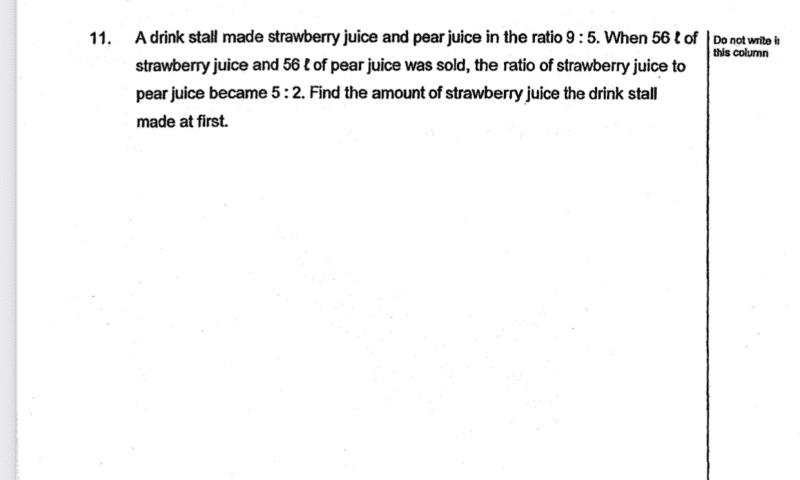QuestionAs same amount (56l) of juices were sold, the difference remain unchanged.

Before:                              S                  :                       P                                Difference

9u                :                       5u                                    4u

Change:                           -56l              :                       -56l                                   0

After:                                5p                :                        2p                                    3p

Since difference unchanged, 4u = 3p (diff). Make them the same by changing to common multiple (i.e. 12)

Before:                              S                      :                       P                                Difference

9ux3                :                       5ux3                                    4ux3

27                                             15                                         12

Change:                           -56l                  :                       -56l                                   0

After:                                5px4                :                        2px4                                    3px4

20                                                8                                          12

Hence, 27-20=7u (for S).

7u = 56l

1u = 8l

Thanks for the help.

0 Replies 0 Likes

S:P  : D       S:P: D
9:5  :4        5:2:3
27:15:12   20:8:12

12u-> 56l
1u->56÷12=14/3
27u->14/3 x 27=126

I don’t know if this is correct but this is my way of doing it 🙂

Sorry I only know how to solve this by algebra…

Let S=Number of strawberries at the beginning
Let P=Number of pears at the beginning

So, S/P = 9/5
=> (1) P = 5/9S

Also after selling,

(S-56)/(P-56)  =5/2

=> 2S – 112 =5P – 280
=> (2) 2S -5P = -168

Sub (1) into (2),

2S – 5(5/9)S = -168
-7/9S = -168
=> S = 168 * 9/7 = 216 #

0 Replies 2 Likes